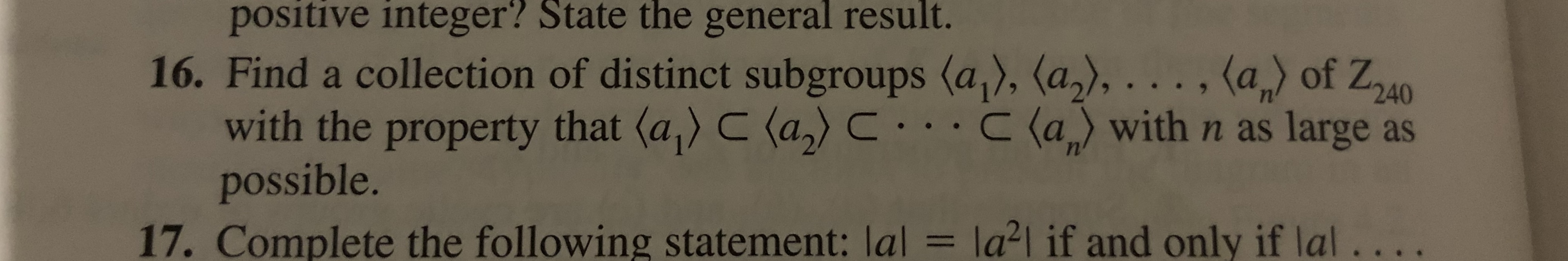positive integer? State the general result.16. Find a collection of distinct subgroups (a), (a2),..., (a of Z40with the property that (a) C (a)C..C (a with n as large aspossible.= la2l if and only if lal..17. Complete the following statement: lal

Question

16help_outlineImage Transcriptionclosepositive integer? State the general result. 16. Find a collection of distinct subgroups (a), (a2),..., (a of Z40 with the property that (a) C (a)C..C (a with n as large as possible. = la2l if and only if lal.. 17. Complete the following statement: lal fullscreen
Step 1

It is known that in the group Zn , if  d divides n , then Zn has a unique subgroup of order d and that subgroup is  <n/d> .

Step 2

Since, the divisors of 240 are {1,2,3,4,5,6,……80,120,240},

Therefore, collection of subgroups of Z240 will be:

<0> = <0>

<120> is a subgroup of order 2.

<80> is a subgroup of order 3.

<60> is a subgroup of order 4.

….          ...

Want to see the full answer?

See Solution

Want to see this answer and more?

Our solutions are written by experts, many with advanced degrees, and available 24/7

See Solution
Tagged in

Math